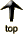Italicized links open a new window to an external site

Your "Inflationary Dollar," how much is it worth today?

Imagine that on the day you were born, your parents placed a dollar bill in a magic box.  The magical properties of this box change the contents to match the changes in the Consumer Price Index.   When the CPI increases, the amount of money in the box decreases because inflation erodes its value.  Likewise when the CPI decreases, the amount in the box increases as deflation increases its value.  Given the changes in the CPI since you were born, how much money do you think you'd find in your magic box if you opened it today?

• You can calculate a manual solution as follows.

The solution results from this equation.

 birth year CPI X ----------------------- = --------- current year CPI \$1

Solving for X tells us what the purchasing power of today's dollar would be in the year of the person's birth.  For example,  I was born in 1947.  The CPI (1982-84=100) stood at 22.3.  As I type, it is December 1998.  The CPI for the year through November is 162.9.  Therefore, X = 22.3/162.9 or .13689.  Rounded off that is 14 cents.

Reversing the equation (finding what \$1 from the person's birth year would purchase today), you see

 birth year CPI \$1 ----------------------- = --------- current year CPI X

For easier reading, we can express the equation as

 current year CPI ----------------------- = X birth year CPI

Solving this for my birth year we find that a 1947 dollar would purchase \$7.30 worth of goods at the end of November 1998. In other words, its value is 1/7.3 of what it was. This (rounded) divides out to same 14 cents we calculated with the first equation. Update: In 2011, the value of my dollar has shrunk to 10 cents.

• If you want to automatically calculate a solution online, you can use the CPI Calculation Machine provided by the Federal Reserve Bank of Minneapolis.   You'll need to enter the current year on the first line, and the person's birth year in the first space on the second.  Leave \$1 in the second space on the first line.  Press the Calculate button, and the result will appear in the second space on the second line.  (The Federal Bureau of Labor Statistics has also posted an online inflation calculator.)

In addition to the calculator, the Minneapolis site gives you a list of the yearly CPI numbers back to 1913.

• The CPI is not the only measure of inflation.  Sam Williamson's How Much Is That? gives us calculators to illustrate the GDP deflator, the Unskilled Wage Rate, the per capita GDP, and as a fraction of the GDP in addition to the CPI.

• "Measuring worth is a complicated question." Find out how one goes about doing it at MeasuringWorth.com

• How much does it cost to raise a child?

Each year since 1960, the US Department of Agriculture issues a report showing the average cost of raising a child in the United States from birth to 17 years.  If you have the Adobe Acrobat Reader, you can read each of those issued since 1996 by clicking here.  If you just want to look at the press releases announcing each of these reports, use the following links.